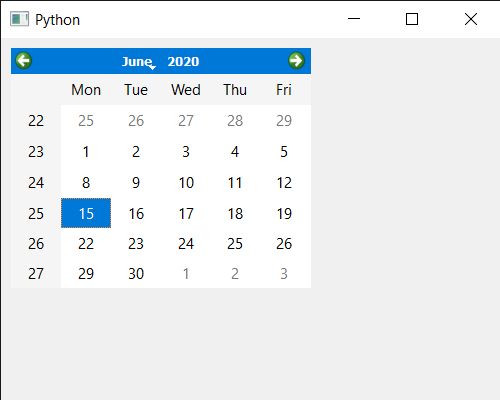Open in App
Not now

# PyQt5 QCalendarWidget – Setting Move Event

• Last Updated : 17 Jun, 2020

In this article we will see how we can implement the move event of QCalendarWidget. This event handler can be reimplemented in a subclass to receive calendar move events which are passed in the event parameter. When the calendar receives this event, it is already at the new position.

Below is the code for the Calendar class

```# QCalendarWidget Class
class Calendar(QCalendarWidget):

# constructor
def __init__(self, parent=None):
super(Calendar, self).__init__(parent)
self.setMouseTracking(True)

# override the move event
def moveEvent(self, event):
print("Calendar Moved")
```

Implementation steps:
1. Create a Calendar class that inherits the QCalendarWidget
2. Inside the Calendar class override the moveEvent and inside the event print the text
3. Create a main window class
4. Create a Calendar object inside the main window
5. Set various properties to the calendar

Below is the implementation

 `# importing libraries``from` `PyQt5.QtWidgets ``import` `*` `from` `PyQt5 ``import` `QtCore, QtGui``from` `PyQt5.QtGui ``import` `*` `from` `PyQt5.QtCore ``import` `*` `import` `sys`` ` `# QCalendarWidget Class``class` `Calendar(QCalendarWidget):`` ` `    ``# constructor``    ``def` `__init__(``self``, parent ``=` `None``):``        ``super``(Calendar, ``self``).__init__(parent)``        ``self``.setMouseTracking(``True``)`` ` `    ``# override the move event``    ``def` `moveEvent(``self``, event):``        ``print``(``"Calendar Moved"``)`` ` ` ` `class` `Window(QMainWindow):`` ` `    ``def` `__init__(``self``):``        ``super``().__init__()`` ` `        ``# setting title``        ``self``.setWindowTitle(``"Python "``)`` ` `        ``# setting geometry``        ``self``.setGeometry(``100``, ``100``, ``500``, ``400``)`` ` `        ``# calling method``        ``self``.UiComponents()`` ` `        ``# showing all the widgets``        ``self``.show()`` ` ` ` `    ``# method for components``    ``def` `UiComponents(``self``):`` ` `        ``# creating a QCalendarWidget object``        ``# as Calendar class inherits QCalendarWidget``        ``self``.calendar ``=` `Calendar(``self``)`` ` `        ``# setting cursor``        ``self``.calendar.setCursor(Qt.PointingHandCursor)`` ` `        ``# setting size of the calendar``        ``self``.calendar.resize(``300``, ``240``)`` ` `        ``# move the calendar``        ``self``.calendar.move(``10``, ``10``)`` ` ` ` ` ` ` ` `# create pyqt5 app``App ``=` `QApplication(sys.argv)`` ` `# create the instance of our Window``window ``=` `Window()`` ` `# start the app``sys.exit(App.``exec``())`

Output :

`Calendar Move`My Personal Notes arrow_drop_up